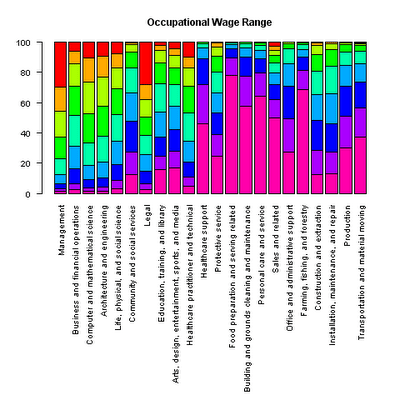Want to share your content on R-bloggers? click here if you have a blog, or here if you don't.

Ever have conversations with your kids about what they are going to do with their life? Still trying to figure out what you are going to do with yours?

Best to not starve…The chart above represents the percentage of each occupation that earn a given hourly wage. The range below represents annual salaries from less than $23,920 (11.50 * 20808) to more than$118,560.The wage range for various occupations displayed above can be constructed using R. I ran into a couple of issues that perhaps some of you can fix (or provide alternatives). The data is from the Bureau of Labor Statistics and was last modified May 14, 2010 at the time this was posted.

Started with the usual setup.

library(XML)
url=’http://www.bls.gov/oes/current/distribution_table.htm’

tb = readHTMLTable(url, header=FALSE)$distribution_table So ended up adding these manually. colnames(tb)=c(‘Major occupational group’, ‘Under$11.50′,’$11.50 to$14.49′,’$14.50 to$18.24′,’$18.25 to$22.74′,’$22.75 to$28.74′,’$28.75 to$35.99′,’$36.00 to$45.24′,’$45.25 to$56.99′,’Over \$57.00’)

Assigned the row names based upon the first column labels (then deleted the first column)

rownames(tb)=tb[,1]
tb=tb[-1]

There were percentage signs interspersed in the data, so stripped these out.

tb=sub(‘%’,”,as.matrix(tb))

Problem #2: How can you fit a legend on a plot like this? Each bar represents 100% (and so all bars are the same size).

par(las=2, mar=c(20, 4, 4, 2) + 0.1, cex=0.85)
barplot(t(tb), main=”Occupational Wage Range”, col=rev(rainbow(9)), names.arg=names(tb))

I eventually decided to display the legend separately and copy it out

barplot(t(tb), main=”Occupational Wage Range”, col=’white’, border=NA)
legend(1, 100, colnames(tb), cex=0.8, fill=rev(rainbow(9)));

I have the feeling that the answer to this questions might be best addressed by something more fundamental, like selecting a different way of representing the data.